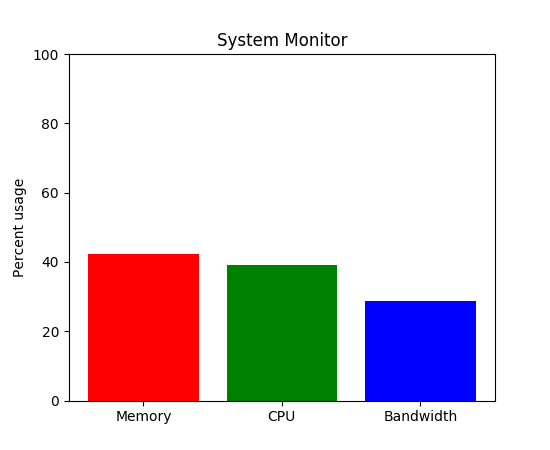Travis-CI:# pylab_examples example code: system_monitor.py¶```import time
import matplotlib.pyplot as plt
import numpy as np

def get_memory(t):
"Simulate a function that returns system memory"
return 100 * (0.5 + 0.5 * np.sin(0.5 * np.pi * t))

def get_cpu(t):
"Simulate a function that returns cpu usage"
return 100 * (0.5 + 0.5 * np.sin(0.2 * np.pi * (t - 0.25)))

def get_net(t):
"Simulate a function that returns network bandwidth"
return 100 * (0.5 + 0.5 * np.sin(0.7 * np.pi * (t - 0.1)))

def get_stats(t):
return get_memory(t), get_cpu(t), get_net(t)

fig, ax = plt.subplots()
ind = np.arange(1, 4)

# show the figure, but do not block
plt.show(block=False)

pm, pc, pn = plt.bar(ind, get_stats(0))
pm.set_facecolor('r')
pc.set_facecolor('g')
pn.set_facecolor('b')
ax.set_xticks(ind)
ax.set_xticklabels(['Memory', 'CPU', 'Bandwidth'])
ax.set_ylim([0, 100])
ax.set_ylabel('Percent usage')
ax.set_title('System Monitor')

start = time.time()
for i in range(200):  # run for a little while
m, c, n = get_stats(i / 10.0)

# update the animated artists
pm.set_height(m)
pc.set_height(c)
pn.set_height(n)

# ask the canvas to re-draw itself the next time it
# has a chance.
# For most of the GUI backends this adds an event to the queue
# of the GUI frameworks event loop.
fig.canvas.draw_idle()
try:
# make sure that the GUI framework has a chance to run its event loop
# and clear any GUI events.  This needs to be in a try/except block
# because the default implementation of this method is to raise
# NotImplementedError
fig.canvas.flush_events()
except NotImplementedError:
pass

stop = time.time()
print("{fps:.1f} frames per second".format(fps=200 / (stop - start)))
```

Keywords: python, matplotlib, pylab, example, codex (see Search examples)